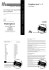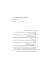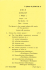# Mathshammil

## Transcription

Mathshammil
```QS016/2
QS016/2
Mathemattcs
Paper 2
Matematik
Kertas 2
I
Semester
Semester
Session 2010/2011
2 hours
I
I
Sesi 2010/2011
2 jam
dL
I
=Y=
a::
l-:--,S
"%XXF"'
BAHAGIAN MATRIKULASI
KEMENTERIAN PELAJARAN MALAYSIA
MAT RIC ULATION DIVISION
MINISTRY OF EDUCATION MALAYSIA
PEPERIKSMN SEMESTER PROGRAM MATRIKULASI
MATRIC ULATION P ROGRAMME EXAMINATION
MATEMATIK
Kertas 2
2 iam
JANGAN BUKA KERTAS SOALAN INISEHINGGA DIBERITAHU.
DO NOT OPEN IHIS BOOKLET UNTILYAU ARE TOLD IO DO SO.
Kertas soalan ini mengandungi 15 halaman bercetak.
Thisbooklet conslsfs
of 15
printedpages.
@ Bahagian Matrikulasi
SHAMMIL
QS016/2
INSTRUCTIONS TO CANDIDATE:
This question booklet consists of 10 questions.
The firll marks for each question or section are shown in the bracket at the end of the question
or section.
A1l steps must be shown clearly.
Only non-programmable scientific calculators can be used.
Numerical answers may be given in the form of fi, e, svrd, fractions or up to three
significant figures, where appropriate, unless stated otherwise in the question.
!7
3
QSo16/2
LIST OF MATHEMATICAL FORMULAE
Differentiation
If y=g(r) *a x=f|\then
!=4"4
dx dt dx
d(dv)l
d'v
dx
dx2-AlA)
dt
Integration
v
ludv=uv- lvdu
rt
E
QS()16/2
1
Find
(a)
!ax
I
foreach of the following:
1 = (ln x)'
.
12
(b)
marl<sl
xy' - ye* =3.
14 marl<s)
2
Find the exact value
of
f
f ,t,'
-t a,
[6 marl<s]
.y
3
If f
is a tunction
with .f '(l) =2, find
lirn"f
GL- f
Q)
x+l riX _1
16
4
Expr.r,
ff{f
Hence, evaluate
as
marksl
partial fractions.
*1t ,r.
!']
J[ 2x'+3x
l7 marl<sl
v
7
QSo16/2
5
f
Given the functions
and
g
as follows:
f(x)=2-*',
8Q) = x +2'
(a)
Find
/.g
and
g.f.
14 marlcs]
(b)
(c)
State the domain and range
of f
"
g.
marla)
12
marksl
Find (g " f)'.
v
(d)
13
Determine the value
of
r
that
such
f
.g(x) = g" f (x).
13 marl<sl
6
(a)
State the conditions of continuity of a function at a
point x = c.
12
(b)
A tunction
/
defined by
I x-21
x'+3x-10
fG)=1 A
v
- -5<.r<2
2<x<3
Ax+B ,
is continuous at x
:2
(i)
/(x).
Find
marksl
lrry_
and x :
x=3
3.
[6 marlrs)
(ii)
Determine the values
of the constants A
and B.
[5 marl<s)
I
QSo16/2
7
(a)
Evaiuate.
(i)
lt
I
a
+zx-L
!+-{
lim
r+€
x+1
13 marl<s)
(ili
rT
.ilil.i 2- Vx'-5
t-.--1
.r + 3
14 morl<sl
-.
/(')(b) If li*
x+{
X-2
5
= 1,
find lim
r-4" /(x).
13 marks)
v
8
Consider the curve given by the equation .f (x) = 2 - x'
(a)
Sketch the region bounded by the curves -f (x)
x
: 0 and x:2.
.
, g(x) = x2, the lines
Hence, find the area of the region.
l7 marks)
(b)
Find the volume of solid generated when the region bounded by the curve
/(x), lines x=1
and
x=2
15 marl<s)
Y
11
QS016/2
9
Consider the parametric equations
x=2t-t-' ,
(a)
!=2tlt'' ,
/>1.
Show that
dy
dx
2r2
-1
2r:
-l
13 marl<sl
(b)
Er aluate
+
(L\
at the
point (1, 3).
14 marks)
Y
(c)
pin6 4! in term of r. Hence, show that
dx'
dry g
=
dx2 Y3'
16
-
13
marksl
QS016/2
10
Atunction
(a)
/
isdefinedby
R.
f(x)="' x'+x
:"- '
5 Y2 -r-
-r-
4
'
Find the vertical and horizontal asymptotes
of /.
13 marl<s)
(b)
Find the coordinates of,the point where the curve
/
cuts the horizontal
asymptote.
[2 marlcs]
(c)
Determine the coordinates of the point where
/'(x)
=
Q.
13
(d)
By writing y
marksl
:f (x), show that
(y-5)r'+(f-8)x-4=0
Hence, for real x, show that
f (x) < -4
or
f (x)>4.
14 marl<s)
(e)
Sketchthe graph
of f
.
13 marl<s)
END OF QUESTION BOOKLET
15
```

### BAHAGIAN MATRIKULASI MATEMATIK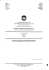### MiniScore MiniScore MiniScore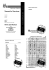### BAHAGIAN MATRIKULASI MATEMATIK CHOW CHOON WOOI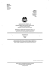### MiniScore MiniScore MiniScore